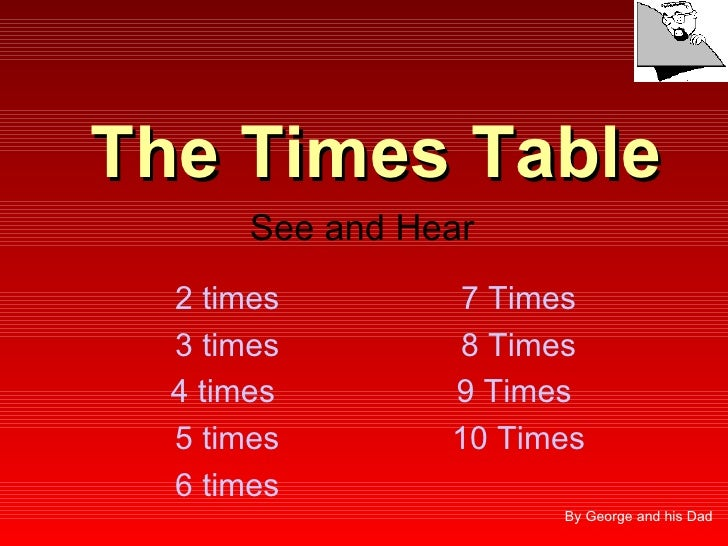Successfully reported this slideshow.Upcoming SlideShare
×

# Times Table

13,266 views

Published on

A slideshow I put together several years ago to help my son learn his times tables. Download to hear the Sound files.

Published in: Education, Spiritual, Technology
• Full Name
Comment goes here.

Are you sure you want to Yes No• Most surveys don't pay cash, ☺☺☺ https://t.cn/A6ybK3XL

Are you sure you want to  Yes  No
• Hey buddy. I actually have been looking for something like this for the longest time. Glad I found out about you guys And also thanks for all the help with my router. ➣➣➣ https://url.cn/ktFCrsHZ

Are you sure you want to  Yes  No
• Hello! I can recommend a site that has helped me. It's called HelpWriting.net They helped me for writing my quality research paper on diabetes, and of course by keeping my all other needs fulfilled.

Are you sure you want to  Yes  No
• Sex in your area is here: ♥♥♥ http://bit.ly/39pMlLF ♥♥♥

Are you sure you want to  Yes  No

Are you sure you want to  Yes  No

### Times Table

1. 1. The Times Table See and Hear 2 times 7 Times 3 times 8 Times 4 times 9 Times 5 times 10 Times 6 times By George and his Dad
2. 2. 2x1=2
3. 3. 2x2=4
4. 4. 2x3=6
5. 5. 2x4=8
6. 6. 2 x 5 = 10
7. 7. 2 x 6 = 12
8. 8. 2 x 7 = 14
9. 9. 2 x 8 = 16
10. 10. 2 x 9 = 18
11. 11. 2 x 10 = 20
12. 12. 3x1=3
13. 13. 3x2=6
14. 14. 3x3=9
15. 15. 3 x 4 = 12
16. 16. 3 x 5 = 15
17. 17. 3 x 6 = 18
18. 18. 3 x 7 = 21
19. 19. 3 x 8 = 24
20. 20. 3 x 9 = 27
21. 21. 3 x 10 = 30
22. 22. 4x1=4
23. 23. 4x2=8
24. 24. 4 x 3 = 12
25. 25. 4 x 4 = 16
26. 26. 4 x 5 = 20
27. 27. 4 x 6 = 24
28. 28. 4 x 7 = 28
29. 29. 4 x 8 = 32
30. 30. 4 x 9 = 36
31. 31. 4 x 10 = 40
32. 32. 5x1=5
33. 33. 5 x 2 = 10
34. 34. 5 x 3 = 15
35. 35. 5 x 4 = 20
36. 36. 5 x 5 = 25
37. 37. 5 x 6 = 30
38. 38. 5 x 7 = 35
39. 39. 5 x 8 = 40
40. 40. 5 x 9 = 45
41. 41. 5 x 10 = 50
42. 42. 6x1=6
43. 43. 6 x 2 = 12
44. 44. 6 x 3 = 18
45. 45. 6 x 4 = 24
46. 46. 6 x 5 = 30
47. 47. 6 x 6 = 36
48. 48. 6 x 7 = 42
49. 49. 6 x 8 = 48
50. 50. 6 x 9 = 54
51. 51. 6 x 10 = 60
52. 52. 7x1=7
53. 53. 7 x 2 = 14
54. 54. 7 x 3 = 21
55. 55. 7 x 4 = 28
56. 56. 7 x 5 = 35
57. 57. 7 x 6 = 42
58. 58. 7 x 7 = 49
59. 59. 7 x 8 = 56
60. 60. 7 x 9 = 63
61. 61. 7 x 10 = 70
62. 62. 8x1=8
63. 63. 8 x 2 = 16
64. 64. 8 x 3 = 24
65. 65. 8 x 4 = 32
66. 66. 8 x 5 = 40
67. 67. 8 x 6 = 48
68. 68. 8 x 7 = 56
69. 69. 8 x 8 = 64
70. 70. 8 x 9 = 72
71. 71. 8 x 10 = 80
72. 72. 9x1=9
73. 73. 9 x 2 = 18
74. 74. 9 x 3 = 27
75. 75. 9 x 4 = 36
76. 76. 9 x 5 = 45
77. 77. 9 x 6 = 54
78. 78. 9 x 7 = 63
79. 79. 9 x 8 = 72
80. 80. 9 x 9 = 81
81. 81. 10 x 1 = 10
82. 82. 10 x 2 = 20
83. 83. 10 x 3 = 30
84. 84. 10 x 4 = 40
85. 85. 10 x 5 = 50
86. 86. 10 x 6 = 60
87. 87. 10 x 7 = 70
88. 88. 10 x 8 = 80
89. 89. 10 x 9 = 90
90. 90. 10 x 10 = 100
91. 91. YOU ARE FINISHED Well Done